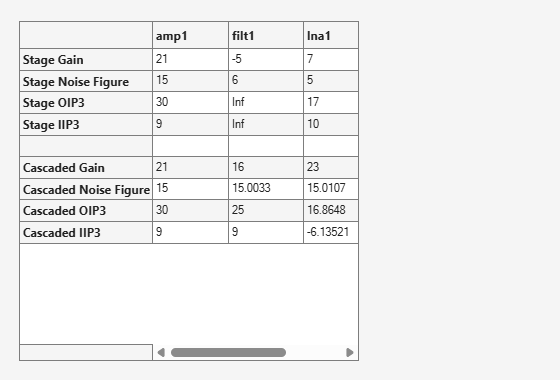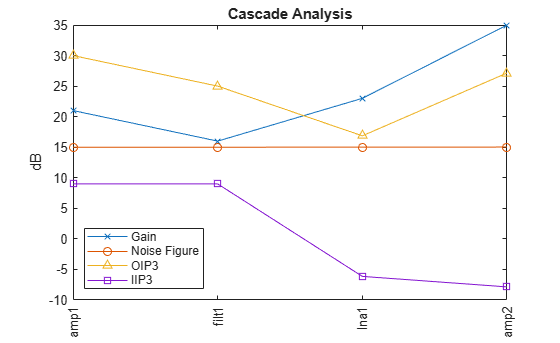# rfchain

Create `rfchain` object

## Description

Use the `rfchain` object to create an RF circuit cascade analysis object to calculate gain, noise figure, OIP3 ( output third-order intercept), and IIP3 (input third order intercept).

## Creation

### Syntax

``rfobj = rfchain()``
``````obj = rfchain(g, nf, o3, 'Name', nm)``````
``````obj = rfchain(g, nf, 'IIP3', i3, 'Name', nm)``````

### Description

example

````rfobj = rfchain()` creates an RF chain object `obj` having zero stages. To add stages to the RF chain, use `addstage` method.```

example

``````obj = rfchain(g, nf, o3, 'Name', nm)``` creates an RF chain object `obj` having `N ` stages. The gain `g`, noise figure `nf` and the OIP3 `o3` are vectors of length `N `. The name `nm` is a cell array of length ```N ```.```
``````obj = rfchain(g, nf, 'IIP3', i3, 'Name', nm)``` creates an RF chain object having `N` stages, specifying an IIP3 for each stage, instead of an OIP3.```

## Properties

expand all

Number of stages in an RF chain, specified as a scalar.

Data Types: `double`

Name of each stage of an RF chain, specified as a character vector. This will always be a name-value pair.

Data Types: `char` | `string`

Gain, in dB, of each stage in an RF chain, specified as a vector.

Data Types: `double`

Noise figure, in dB, of each stage in an RF chain, specified as a vector.

Data Types: `double`

Output-referred third-order intercept, in dB, of each stage in an RF chain, specified as a vector.

Data Types: `double`

Input-referred third-order intercept, in dB, of each stage in an RF chain, specified as a vector.

Data Types: `double`

## Examples

collapse all

Create an RF chain object.

`rfch = rfchain;`

Add stage 1 and stage 2 with gain, noise figure, oip3.

```addstage(rfch, 21, 15, 30, 'Name', 'amp1'); addstage(rfch, -5, 6, Inf, 'Name', 'filt1');```

Add stage 3 and stage 4 with gain, noise figure, iip3.

```addstage(rfch, 7, 5, 'IIP3', 10, 'Name', 'lna1'); addstage(rfch, 12, 14, 'IIP3', 20, 'Name', 'amp2');```

Calculate the gain, noise figure, oip3, and iip3 of each stage.

```g = cumgain(rfch); nf = cumnoisefig(rfch); oip3val = cumoip3(rfch); iip3val = cumiip3(rfch);```

View the results on a table and plot it.

`worksheet(rfch)````figure plot(rfch)```Assign three stage-by-stage values of gain, noise figure, OIP3 and stage names.

```g = [11 -3 7]; nf = [25 3 5]; o3 = [30 Inf 10]; nm = {'amp1','filt1','lna1'};```

Create an RF chain object.

`rfch = rfchain(g,nf,o3,'Name',nm);`

View results in a worksheet.

`worksheet(rfch)`## Version History

Introduced in R2013b